### Conservation of Energy

The first thing we must do is define our terms.  Force, energy, and power are all related to each other in very specific ways.  Remembering that acceleration is defined as the rate of change of the velocity, or a = v / t, gives us the following table:

 Quantity Definition Units Unit Name Abbreviation force = ma kg m/s2 newton N energy = Fd kg m2/s2 joule J power = E/t kg m2/s3 watt W

Or put into words, energy is force acting through a distance, and power is the energy expended per second.  A joule is the amount of energy needed to exert a force of one newton through one meter.  For example, if you lift a one-kilogram weight one meter, then the energy you must expend is:

E = Fd = (ma)d = mgd = (1 kg)(9.8m/s2)(1 m) = 9.8 kg m2/s2 = 9.8 joules.

An alternative definition of energy, the calorie, is defined as the amount of heat energy needed to raise the temperature of 1 gm of water 1 C°.  (There was a time when folks didn't realize that heat energy and mechanical energy are really one and the same, so they created separate definitions for them.)  Thermodynamic measurements tell us that one calorie = 4.186 joule.
By the way -- the "calorie" commonly used in measuring food energy is actually the kilocalorie, or Kcal for short.  One Kcal = 1000 cal = 4168 joules.  So, for example, a Hostess Twinkie with 200 calories contains 200 X 4168 = 833,600 joules, or enough energy to raise a 100-kilogram weight over half a mile!  (Now you know why Twinkies are not normally considered to be diet food.)
Rather than using joules, the "kilowatt-hour" is commonly used by the electrical industry as a unit of energy.  (The kw-hr is NOT a unit of power.  Despite having the power-term "watt" in its name, the kw-hr is a unit of energy.  Since power = energy / time, it is also true that energy = power X time.)  A kw-hr is a convenient quantity in the electric industry, because it is the energy equivalent of running a one-kilowatt appliance for one hour.  The conversion of a kilowatt-hour into joules is just:

kilowatt-hour = (1000 J/s)(1 hr) X (60 min/hr)(60 sec/min) = 3,600,000 J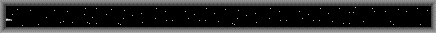Mysterious form of alien energy?

Forms of Energy

Energy is a mysterious concept, not least because it comes in many guises.  Here is a listing of the common forms:

kinetic energy -- the energy of moving bodies.  The formula for kinetic energy is:
E = ½ mv2
elastic energy -- the energy contained in stretched or strained materials.  Rubber bands, springs, anything with tension on it, etc.  For a simple spring, the formula for elastic energy is:
E = ½ K(x - x0)2

where K = the spring constant (units of N / m), x0 = the equilibrium position of the spring, and x = the distance the spring has been stretched or compressed.

thermal energy -- also known as heat.  This is more-or-less a special case of kinetic energy and elastic energy, because what we call thermal energy consists of the energy of many atoms.  In the case of a gas, where the molecules are moving freely, the average kinetic energy per molecule can be related to the absolute temperature by:
½ mv2 = 3/2 kBT

where m = the mass of the gas molecule (we assume the gas contains only one kind of molecule), v = the average velocity of the molecules, kB = Boltzmann's constant = 1.38 X 10-23 J/K°, and T = the temperature in K°.
For a solid or liquid of mass m, the change in heat energy can be related to the change in temperature by:
dQ = mc dT

where dQ = the change in heat, c is the specific heat capacity in cal/gm K°, and dT = the change in temperature (in either C° or K°).
chemical energy -- the energy associated with breaking or making atomic bonds, i.e., with chemical transformations.

latent heat -- the energy needed to melt solids or boil liquids.  This energy is somewhat similar to chemical energy, since it is the energy associated with breaking or making molecular bonds, rather than atomic bonds.  The total energy (heat) needed to melt a solid or boil a liquid is just Q = mL, where m is the mass of the liquid or solid, and L is the latent heat factor (given in either J or cal per kg) of melting or boiling.

gravitational potential energy -- the energy acquired or lost by bodies as they are moved within a gravitational field.  For objects very near the Earth, the gravitational potential energy is given by E = mgh, where m is the mass of the object, g is the acceleration of gravity (9.8 m/s2) and h is the height to which the object is raised.

electromagnetic energy -- the energy contained within electromagnetic fields.  Common examples include household electricity, the field around a magnet, and static electricity.  For household electricity, the energy dissipated by a circuit is E = IVt, where I is the current (in amps), V is the voltage, and t is the time (in seconds) that the current flows.

radiant energy -- the energy of radio waves, microwaves, infrared light, visible light, UV light, x-rays, and gamma-rays.  Everything gives off radiant energy, to some degree.  The total amount of energy given off as radiant energy by any object is approximately:
E = SA(T - T0)4

where S = the Stefan-Boltzmann constant = 5.67 X 10-8 W/m2 °K4
A = the surface area of the object
T = the absolute temperature of the object
T0 = the absolute temperature of the object's surroundings
The above formula only works if T is greater than T0; otherwise, the object will absorb radiant energy from its surroundings, since heat always flows from hot to cold.

nuclear energy -- the energy associated with radioactivity and nuclear interactions.  This is quantified by the simple but profound formula E = mc2, which tells us that mass itself is only another form of energy.

Historically, it wasn't until roughly the mid-1800's that physicists realized that all forms of energy can be transmuted into each other.  Heat energy was thought to be a kind of fluid, called "caloric", which flowed from one place to another like water.  Kinetic energy was viewed as an entirely separate thing (and was often confused with linear momentum).  Light....well, people weren't quite sure even what light was, never mind how it fit into the energy question.

It was in 1798 that Count Rumford demonstrated that the caloric theory of heat must be wrong.  Rumford was in charge of boring out cannons for the Prussian military, and he had noticed that the water necessary for cooling the cannons as they were bored out continued to be heated even after the drill tips had become so blunt that they weren't cutting into the metal anymore.  The caloric theory said that drilling the metal squeezed out caloric fluid from the small shavings, which is why the cannons got hot -- but Rumford realized that this explanation was impossible.  He set up a public demonstation where blunt rods were rotated against flat metal for hours on end, eventually creating so much heat that the cooling water began to boil -- but there were no shavings being created.  Rumford stated that motion alone was enough to create heat, i.e., he realized that mechanical energy and thermal energy must be two aspects of the same thing.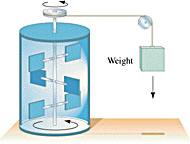James Joule measured the equivalency of mechanical and thermal energy in 1843 (i.e., he determined that one cal = 4.186 J) by examining how much the water in highly insulated calorimeters was heated as it was agitated by paddles driven by falling weights.  The basic idea of his experiment is shown at right.  In 1847, from this experiment and other considerations, Hermann Helmholtz enunciated the Principle of Conservation of Energy.  To put it succinctly:

Energy is Conserved

All the different forms of energy (such as those listed above) may be transmuted into each other or transferred from one place to another, but the total energy in the Universe always remains the same.  Allow me to repeat myself, because this is one of the most important principles in physics:

The total energy of the Universe is constant.
Energy can never be created or destroyed.
Energy can only be moved or transformed.

Energy is Mother Nature's equivalent of money.  Anything you want to do (walk across the room?  power up the stereo?  throw a baseball?  blow up a safe?) requires energy money.  You can spend it however you want, but if you spend it, something else gains it.  There are no profits in Mother Nature, and no losses.  Unlike that paper stuff the Federal government prints, energy money is indestructible and cannot be counterfeited.

It is pretty easy to switch from one currency to another, though.  Just look at your car.  First nuclear energy in the Sun is converted into radiant electromagnetic energy, then plants on Earth convert the radiant energy to chemical energy (oil), then you start your car and convert that into kinetic energy, and finally you hit the brakes, which use friction to convert the kinetic energy into heat.

Human beings mostly get their energy from the Sun.  Sunlight is absorbed by plants and turned into organic molecules, i.e., they convert the radiant energy into chemical energy.  When you hear the exercise gurus talking about "burning calories", that is exactly correct.  Humans turn the chemical energy they eat into body heat and kinetic energy by literally burning sugars, carbohydrates, and fats.
Amusing aside -- they determine the calorie content of foods by placing them in insulated ovens, and setting fire to the food.  After the food has been burned to a pile of fine black ash, they measure the overall temperature of the oven.  Since they know exactly how much energy they introduced into the oven to burn the food, any additional temperature increase can only have come from the burning food itself.  This is a perfectly valid way to do the measurement, even though the human body (as you may have noticed) does not burn its calories in a single, uncontrolled conflagaration.  Rather, it generates heat via millions of little molecule-sized "fires" spread throughout the body.

But either way, you are releasing the same amount of energy.  Imagine heating a bottle of water from the inside, via millions of tiny sparks flashing inside the water, and you have a pretty good picture of how humans maintain their body heat without burning their food in a single fire like they do in calorie-content measurements.
Most of our society's energy needs are provided by gas, oil, and coal.  These are the highly compressed and chemically fermented remains of forests which grew millions of years ago.  (Gas and oil are really fossilized sunlight, when you get down to it.)  Many other forms of energy, such as hydroelectric power and wind power, also derive from the Sun, because it is solar heating which drives the weather and creates the rains that fill the hydroelectric dams.  In fact, the only types of energy used on Earth that don't come from the Sun are geothermal energy, nuclear energy, and hydroelectric energy that is generated by the tides rather than rainfall.  All of these together make up only an insignificant sliver of humanity's total energy use.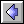Newton's Laws  |  Conservation Laws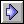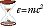Ideas of Physics Homepage

Translation to other languages: Ukrainian, Estonian